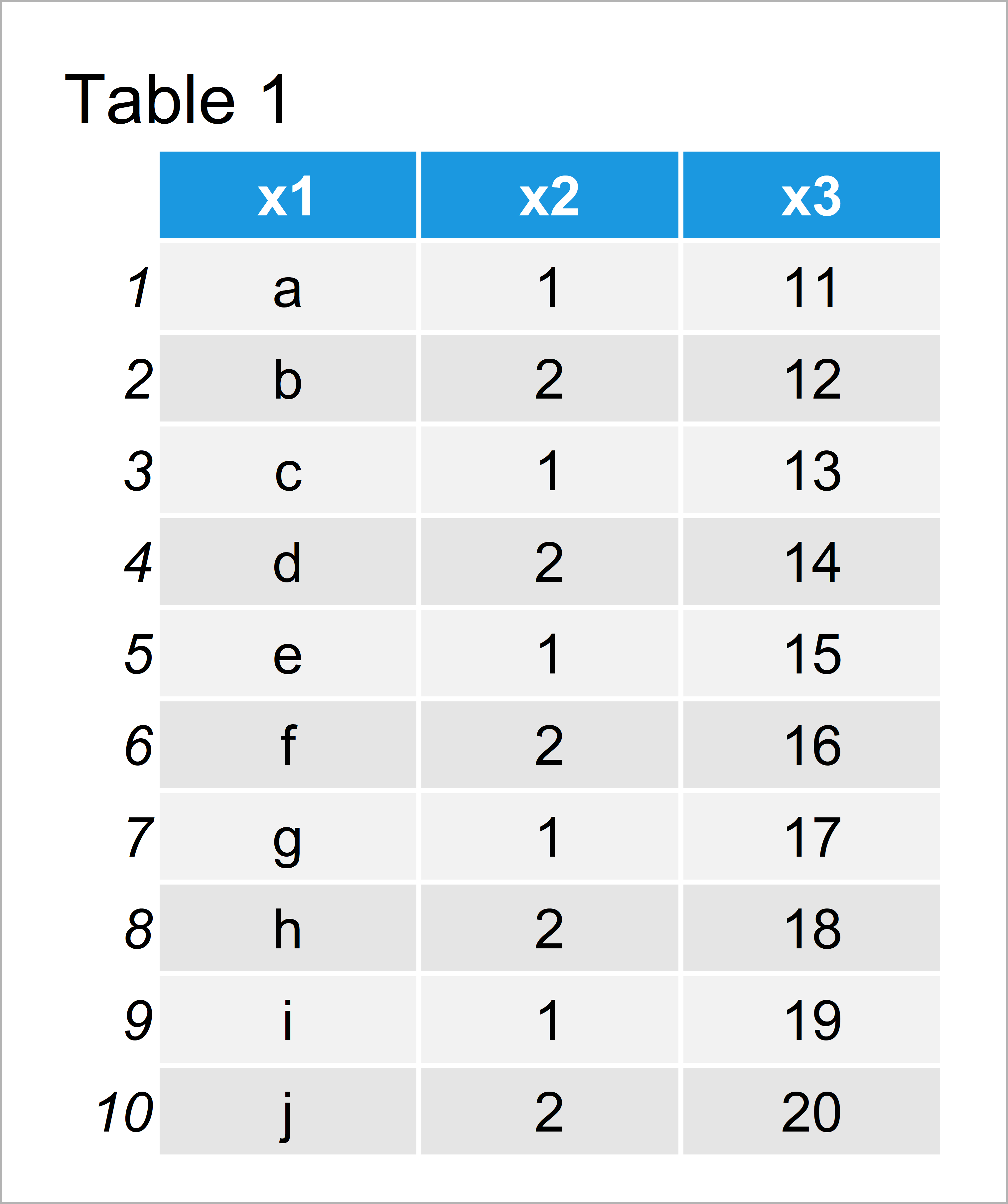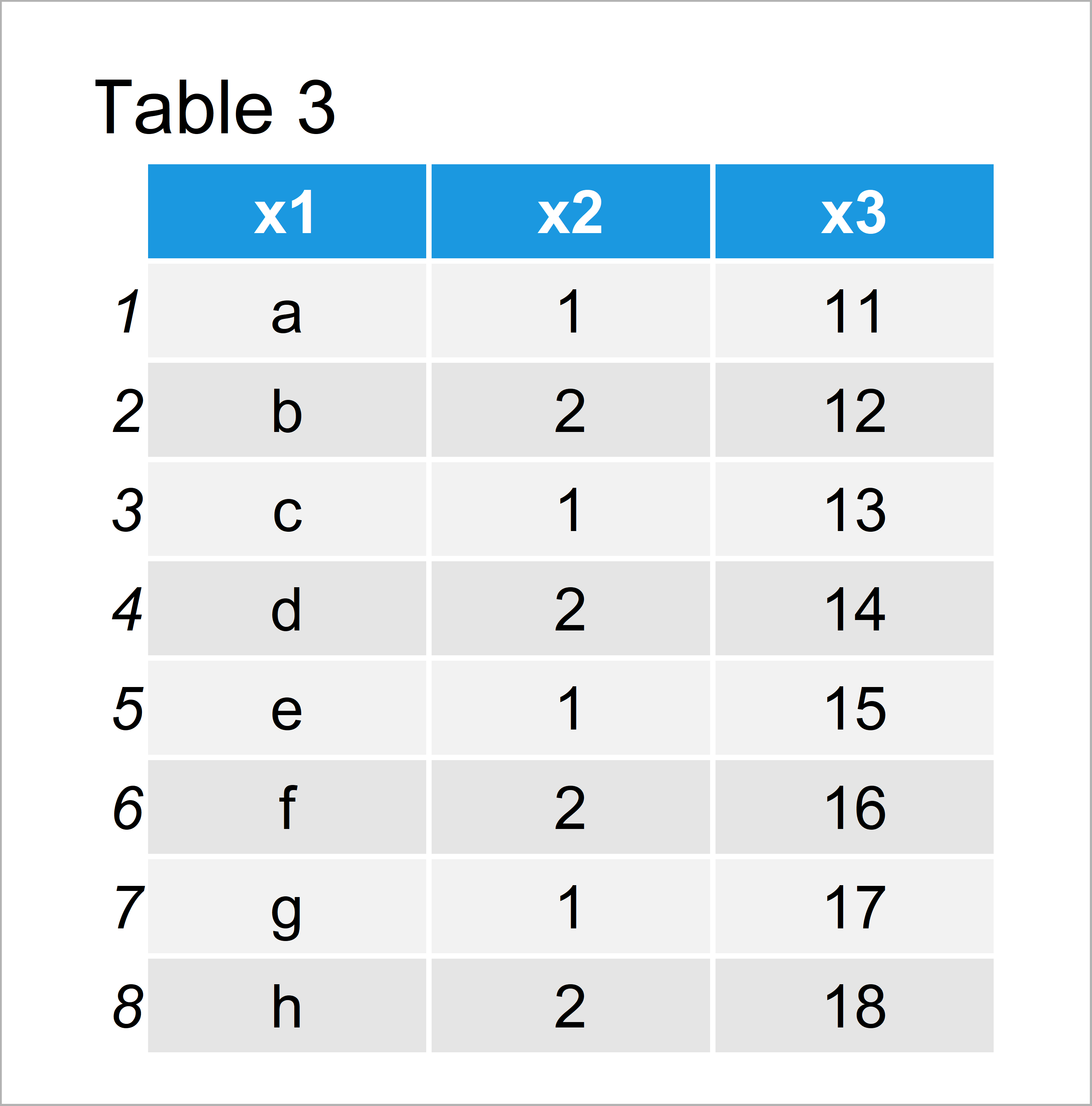# Print First or Last Rows of Data Frame in R (4 Examples)

In this R programming tutorial you’ll learn how to return the first or last n rows of a data frame.

The page is structured as follows:

It’s time to dive into the examples…

## Constructing Example Data

We’ll use the data below as basement for this R programming language tutorial:

```data <- data.frame(x1 = letters[1:10],    # Create example data
x2 = 1:2,
x3 = 11:20)
data                                      # Print example data```As you can see based on Table 1, our example data is a data frame consisting of ten rows and three columns.

## Example 1: Returning First Six Rows of Data Frame Using head() Function

In this example, I’ll explain how to show only the first six rows of our data frame in the RStudio console.

For this, we can apply the head function as shown below:

`head(data)                                # Return first six rows`Table 2 illustrates the output of the previous R code: It shows the first six rows of our data frame.

## Example 2: Returning First N Rows of Data Frame Using head() Function

In this example, I’ll explain how to use the head function to return basically any number of rows from the top of our data frame.

To do this, we have to specify a numeric value indicating the number of rows we want to extract within the head function:

`head(data, 8)                             # Return first eight rows`As you can see based on Table 3, we have printed the first eight rows of our data frame to the RStudio console.

## Example 3: Returning Last Six Rows of Data Frame Using tail() Function

We can use the tail function to do the opposite of the head function, i.e. returning the bottom of our data frame.

Have a look at the following R code:

`tail(data)                                # Return last six rows`As you can see in Table 4, we have extracted the last six rows of our data matrix.

## Example 4: Returning Last N Rows of Data Frame Using tail() Function

As with the head function, we can also specify a particular number of rows that we want to extract from the tail of our data frame.

Consider the following R syntax:

`tail(data, 8)                             # Return last eight rows`The previously shown R code has returned the last eight rows of our data table.

## Video, Further Resources & Summary

I have recently released a video on my YouTube channel, which illustrates the examples of this post. You can find the video below:

In addition, you may have a look at some related articles of this website.

In this article, I illustrated how to extract the top or the bottom of a data set in the R programming language. In case you have additional questions, let me know in the comments.

Subscribe to the Statistics Globe Newsletter